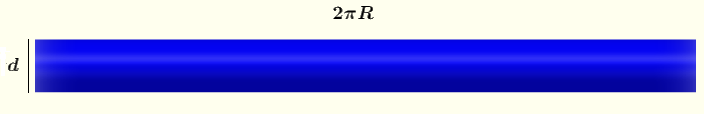# Volume and Surface Area of Torus Calculator

An online calculator to calculate the volume and surface area of a torus ring is presented.

## Formulas for the Volume and Surface Area

A torus ring is generated by rotating a small circle of diameter $d$ along the perimeter of a larger circle.Figure 1. Torus Ring Views of a torus cut along the plane $xy \; (z = 0)$ (on right) and another cut along the plane $yz \; (x = 0)$ (on left) are shown below.
$r_1$ is the inner radius and $r_2$ is the outer radius of the torus.Figure 2. Cuts of Torus Ring A torus cut and developed into a cylinder of diameter $d$ and length $2 \pi R$Figure 3. Torus Ring Developed as a Cylinder

Let
$r_2 = R + d/2$    (I)
$r_1 = R - d/2$    (II)
Add the above equations and simplify to obtain
$r_2 + r_1 = 2 R$
which gives
$R = \dfrac{r_2 + r_1}{2}$
Subtract the two equations (I) and (II) above and simplify top obtain
$r_2 - r_1 = d/2 + d/2 = d$

The volume $V$ of the torus may be calculated as the volume of the cylinder in figure 3. Hence
$V = \pi \left( \dfrac{d}{2} \right)^2 \times 2 \pi R$

Substitute $d$ and $R$ by their expressions in terms of $r_1$ and $r_2$ to obtain
$V = \pi \left( \dfrac{r_2 - r_1}{2} \right)^2 \times 2 \pi \left(\dfrac{r_2 + r_1}{2} \right)$ Simplify to obtain the formula             $\Large \color{red}{V = \dfrac{1}{4} \pi^2 ( r_2 - r_1 )^2 (r_2 + r_1)}$

Using figure 3 above, the lateral surface area $A_L$ of the torus may be calculated as the lateral surface area of the cylinder as follows
$A_L = \pi d \times 2 \pi R$
Substitute $d$ and $R$ by their expressions in terms of $r_1$ and $r_2$ to obtain
$\Large \color{red}{A_L = \pi (r_2 - r_1)(r_2 + r_1)}$

## How to use the calculator

Enter the inner and outer radii of the torus, $r_1$ and $r_2$ respectively, as positive real numbers, with $r_2 > r_1$ and press "calculate". The outputs are volume $V$ and the lateral area $A_L$ of the torus.

 Inner Radius: $r_1$ = 3 Outer Radius: $r_2$ = 5 Number of Decimls = 3